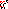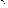# MINLPLib

### A Library of Mixed-Integer and Continuous Nonlinear Programming Instances

#### Instance: house

 Formatsⓘ ams gms lp mod nl osil pip Primal Boundsⓘ -4500.00000000 p1 ( gdx sol ) (infeas: 0) Dual Boundsⓘ -4500.00443600 (ANTIGONE)-4500.00000900 (BARON)-4500.00001100 (COUENNE)-4500.00008100 (LINDO)-4500.00001300 (SCIP) Referencesⓘ Borland, Eureka: The Solver, Borland International, Scotts Valley, California, 1987. Sourceⓘ GAMS Model Library model house Applicationⓘ Geometry Added to libraryⓘ 31 Jul 2001 Problem typeⓘ QCP #Variablesⓘ 8 #Binary Variablesⓘ 0 #Integer Variablesⓘ 0 #Nonlinear Variablesⓘ 5 #Nonlinear Binary Variablesⓘ 0 #Nonlinear Integer Variablesⓘ 0 Objective Senseⓘ min Objective typeⓘ linear Objective curvatureⓘ linear #Nonzeros in Objectiveⓘ 2 #Nonlinear Nonzeros in Objectiveⓘ 0 #Constraintsⓘ 8 #Linear Constraintsⓘ 5 #Quadratic Constraintsⓘ 3 #Polynomial Constraintsⓘ 0 #Signomial Constraintsⓘ 0 #General Nonlinear Constraintsⓘ 0 Operands in Gen. Nonlin. Functionsⓘ Constraints curvatureⓘ indefinite #Nonzeros in Jacobianⓘ 23 #Nonlinear Nonzeros in Jacobianⓘ 9 #Nonzeros in (Upper-Left) Hessian of Lagrangianⓘ 8 #Nonzeros in Diagonal of Hessian of Lagrangianⓘ 0 #Blocks in Hessian of Lagrangianⓘ 1 Minimal blocksize in Hessian of Lagrangianⓘ 5 Maximal blocksize in Hessian of Lagrangianⓘ 5 Average blocksize in Hessian of Lagrangianⓘ 5.0 #Semicontinuitiesⓘ 0 #Nonlinear Semicontinuitiesⓘ 0 #SOS type 1ⓘ 0 #SOS type 2ⓘ 0 Infeasibility of initial pointⓘ 1500 Sparsity JacobianⓘSparsity Hessian of Lagrangianⓘ```\$offlisting
*
*  Equation counts
*      Total        E        G        L        N        X        C        B
*          9        5        3        1        0        0        0        0
*
*  Variable counts
*                   x        b        i      s1s      s2s       sc       si
*      Total     cont   binary  integer     sos1     sos2    scont     sint
*          9        9        0        0        0        0        0        0
*  FX      0
*
*  Nonzero counts
*      Total    const       NL      DLL
*         26       17        9        0
*
*  Solve m using NLP minimizing objvar;

Variables  x1,x2,x3,x4,x5,x6,x7,x8,objvar;

Equations  e1,e2,e3,e4,e5,e6,e7,e8,e9;

e1.. -(x1*x2 + x5*x4) + x7 =E= 0;

e2.. -x1*x3 + x8 =E= 0;

e3..  - x7 - x8 - objvar =E= 0;

e4..  - x2 - x5 + x6 =E= 0;

e5..    x1 - 0.333333333333333*x4 =G= 0;

e6..    x1 - 0.5*x4 =L= 0;

e7.. x2*(x4 - x1) =G= 1500;

e8..  - 0.5*x2 + x3 - x5 =E= 0;

e9..  - 0.5*x2 + x5 =G= 0;

* set non-default bounds
x4.lo = 40; x4.up = 68;
x6.lo = 56; x6.up = 100;
x7.up = 3000;

* set non-default levels
x1.l = 30;
x4.l = 68;

Model m / all /;

m.limrow=0; m.limcol=0;
m.tolproj=0.0;

\$if NOT '%gams.u1%' == '' \$include '%gams.u1%'

\$if not set NLP \$set NLP NLP
Solve m using %NLP% minimizing objvar;

```

Last updated: 2019-02-14 Git hash: a71254dc